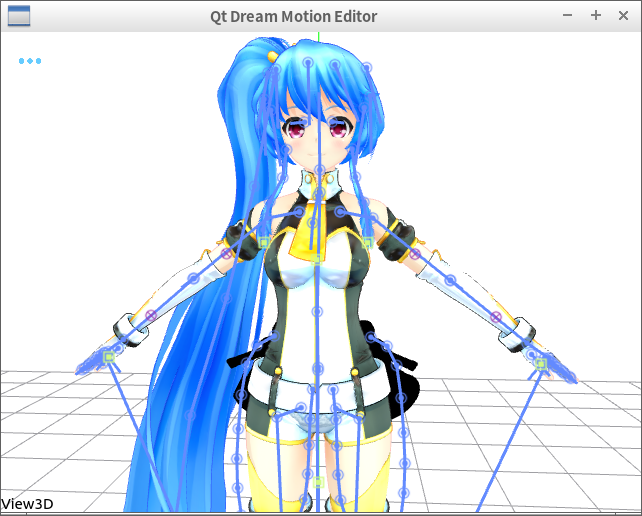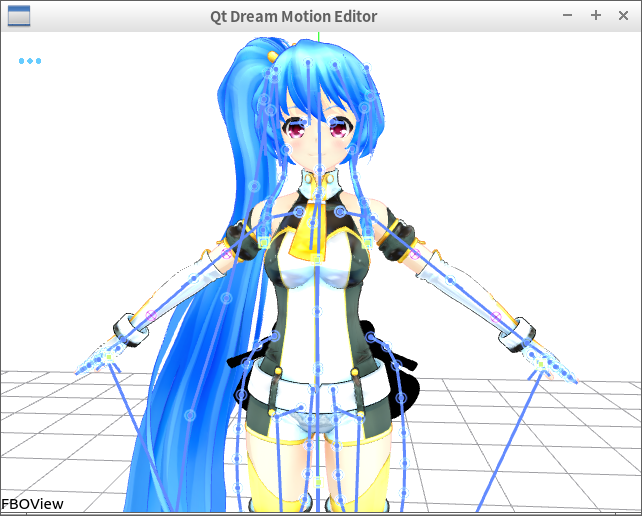# Screen framebuffer VS. QQuickFramebufferObject in texture blending

• I found some difference between default framebuffer provided by screen. Look at this:When I use QQuickFramebufferObject the texture blending is strange:Blending texture is lighter than the default framebuffer.
Could anybody give me a hint on how to cause this difference?

• Which blending mode are you selecting in each version? (If you're not explicitly controlling it, it might default to something different in each case). My first guess would be it's something to do with whether premultiplied alpha is being used or not, just because so many people get tripped up by that.

• @timday Yeah, something must be set before `QQuickWindow::beforeSynchronizing`.
However, I tested so many flags using `glGetIntegerv` but got not much useful information. I use the following code:

``````    GLboolean blend = -1;
glGetBooleanv( GL_BLEND, &blend );
qDebug( ) << "Blend state is: " << ( blend? "enabled": "disabled" );

GLfloat colors;
glGetFloatv( GL_BLEND_COLOR, colors );
qDebug( ) << "Blend color is: " << colors << colors << colors << colors;

GLint equationRGB = -1, equationAlpha = -1;
glGetIntegerv( GL_BLEND_EQUATION_RGB, &equationRGB );
qDebug( ) << "Equation RGB is: " << equationRGB;

glGetIntegerv( GL_BLEND_EQUATION_ALPHA, &equationAlpha );
qDebug( ) << "Equation Alpha is: " << equationAlpha;

glEnable( GL_BLEND ); glBlendFunc( GL_SRC_ALPHA, GL_ONE_MINUS_SRC_ALPHA );

GLint srcRGB = 0, srcAlpha = 0;
GLint destRGB = 0, destAlpha = 0;
glGetIntegerv( GL_BLEND_SRC_RGB, &srcRGB );
glGetIntegerv( GL_BLEND_SRC_ALPHA, &srcAlpha );
glGetIntegerv( GL_BLEND_DST_RGB, &destRGB );
glGetIntegerv( GL_BLEND_DST_ALPHA, &destAlpha );

qDebug( ) << "source RGB is: " << srcRGB <<
"source Alpha is: " << srcAlpha <<
"dest RGB is: " << destRGB <<
"dest Alpha is: " << destAlpha;
glEnable( GL_DITHER );
GLboolean doubleBuffering = -1;
glGetBooleanv( GL_DOUBLEBUFFER, &doubleBuffering );
qDebug( ) << "Double buffering is: " << ( doubleBuffering? "enabled": "disabled" );
``````